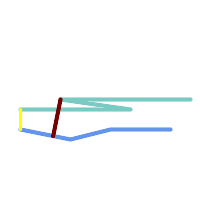## Name

ST_HausdorffDistance — Returns the Hausdorff distance between two geometries.

## Synopsis

`float ST_HausdorffDistance(`geometry g1, geometry g2`)`;

`float ST_HausdorffDistance(`geometry g1, geometry g2, float densifyFrac`)`;

## Description

Returns the Hausdorff distance between two geometries. The Hausdorff distance is a measure of how similar or dissimilar 2 geometries are.

The function actually computes the "Discrete Hausdorff Distance". This is the Hausdorff distance computed at discrete points on the geometries. The `densifyFrac` parameter can be specified, to provide a more accurate answer by densifying segments before computing the discrete Hausdorff distance. Each segment is split into a number of equal-length subsegments whose fraction of the segment length is closest to the given fraction.

Units are in the units of the spatial reference system of the geometries.This algorithm is NOT equivalent to the standard Hausdorff distance. However, it computes an approximation that is correct for a large subset of useful cases. One important case is Linestrings that are roughly parallel to each other, and roughly equal in length. This is a useful metric for line matching.

Availability: 1.5.0

## ExamplesHausdorff distance (red) and distance (yellow) between two lines

```SELECT ST_HausdorffDistance(geomA, geomB),
ST_Distance(geomA, geomB)
FROM (SELECT 'LINESTRING (20 70, 70 60, 110 70, 170 70)'::geometry AS geomA,
'LINESTRING (20 90, 130 90, 60 100, 190 100)'::geometry AS geomB) AS t;
st_hausdorffdistance | st_distance
----------------------+-------------
37.26206567625497 |          20
```

Example: Hausdorff distance with densification.

```SELECT ST_HausdorffDistance(
'LINESTRING (130 0, 0 0, 0 150)'::geometry,
'LINESTRING (10 10, 10 150, 130 10)'::geometry,
0.5);
----------------------
70
```

Example: For each building, find the parcel that best represents it. First we require that the parcel intersect with the building geometry. `DISTINCT ON` guarantees we get each building listed only once. `ORDER BY .. ST_HausdorffDistance` selects the parcel that is most similar to the building.

```SELECT DISTINCT ON (buildings.gid) buildings.gid, parcels.parcel_id
FROM buildings
INNER JOIN parcels
ON ST_Intersects(buildings.geom, parcels.geom)
ORDER BY buildings.gid, ST_HausdorffDistance(buildings.geom, parcels.geom);
```